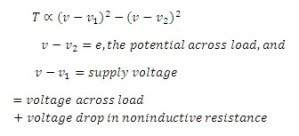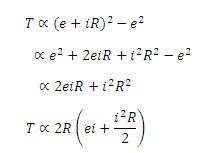# Electrostatic type Wattmeter

## Electrostatic Wattmeter:

These wattmeters are used for measurement of small amount of power, practically when the voltage is high and power factor is low. This type of wattmeter is also used for measurement of dielectric loss of cables on alternating voltage and for calibration of wattmeters and energy meters.

Electrostatic wattmeter consists of a quadrant electrometer used with a non-inductive resistor R as shown in figure below.

Instantaneous torque,Instantaneous torque,i.e. instantaneous torque is proportional to the instantaneous power in the load plus half of the power lost in noninductive resistance.

• Electrostatic wattmeter is a precision instrument and should be used as such.

• It is free from errors on account of waveforms, frequency and eddy currents.

• It has a very small working torque.

You may also like:

Thermal wattmeter

Dynamometer type wattmeter